Chapter 7 – Flip-Flops, and Registers

Chapter Overview

Up to this point we have focused on combinational circuits, which do not have memory.
We now focus on circuits that have “state” or memory.  These are called sequential circuits.  The most basic sequential circuit type that we shall study is called the Flip-Flop; we shall study four different varieties of these devices.  After studying these devices, we consider their use in registers and register files, which can be considered as one form of on–CPU memory.  We note here that conventional memory, called RAM, is normally not on the CPU chip (although a Level 1, or L1, cache always is; and a L2 cache often is).  Conventional RAM and its varieties, including RAM, ROM, SRAM, DRAM, and SDRAM, will be discussed in another chapter.

Chapter Assumptions
Again, the presumption is that the student is familiar with Boolean logic and basic combinational circuitry, specifically NOR gates.  Although many students will have had a previous introduction to flip-flops and latches, no familiarity with the devices is assumed.

Feedback: Asynchronous and Synchronous
Flip-flops belong to one of the simpler classes of sequential circuits.  Basically, a sequential circuit is a circuit that contains memory elements.  Though we shall discuss memory in more detail later, at this point we focus on only one aspect of memory – it can be considered as a form of feedback.  Feedback refers to taking part of the output of a circuit, delaying it for a fixed time period, and then feeding it back as input to the same circuit.  This feedback depends on the time shift caused by the circuit’s gate delays.

There are two basic types of feedback – synchronous and asynchronous.  In synchronous logic, there is a pulse, called the clock pulse that determines when the feedback can be applied to the input.  This clock pulse serves as a fixed time delay on the feedback, and its predictability considerably simplifies digital design.

The following figure shows a deceptively simple circuit with feedback.  In this case, the feedback is asynchronous in that it appears at the NOR gate whenever it is available.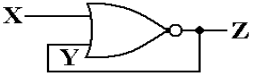We begin the analysis of this circuit by recalling the truth table for a NOR gate.

 X Y X OR Y X NOR Y 0 0 0 1 0 1 1 0 1 0 1 0 1 1 1 0

The important fact to note here is that the output of any NOR gate is 0 when one of its inputs is 1.  In particular, when X = 1 in the above circuit we have Z = 0.  If Z = 0 then
Y = 0, leading again to Z = 0.  When X = 1, the circuit is stable.

Now consider what happens when X = 0 in the above circuit.  If Y = 0 then Z = 1, and if
Y = 1 then Z = 0.  But the circuit requires that Y = Z.  Were it not for the gate delay associated with the NOR gate we would have an impossible situation.

We now present the circuit again and do a detailed timing analysis.  We start with X = 1, which causes Z = 0 and thus Y = 0.  But NOR(1, 0) = 0, so we have a stable circuit.  At some later time, the input X becomes X = 1, and things become interesting.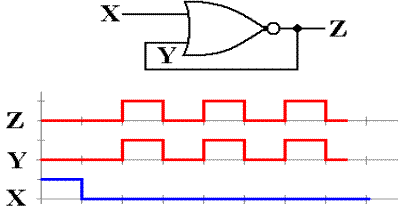With X = 0 and Y = 0, the output Z remains at 0 for one gate delay (approximately ten nanoseconds) and then becomes 1.  Y instantaneously becomes 1 also, as it is connected to Z through a straight wire with a delay of less than 0.001 nanoseconds.  With X = 0 and Y = 1, Z becomes 0 after another gate delay.  Y then becomes 0 and Z changes after another gate delay to Z = 1.  The whole situation is show in the figure just above.

In a moment, we shall turn our attention to the system clock, which is seen as a regular square wave of the sort seen for either Y or Z in the above figure.  Indeed, this circuit could be used for generating a standard clock pulse.  The reason that it is not is probably related to lack of uniformity in the gate delay; measurements for a large number of such circuits might yield gate delays from 9.9 to 10.1 nanoseconds.  Modern electronic units, including all computers, use a crystal oscillator to generate clock pulses at a very precise frequency.  Quartz, being piezoelectric, is the most commonly chosen crystal.

Review of Frequency Measurements
At this time, it might be good to review the notation used to speak of clocks and clock frequencies.  The common unit is a Hertz, named after a physicist who made notable contributions to the theory of electricity and magnetism.  The unit is abbreviated “Hz”.

An event happens at a frequency of 1 Hz if it happens once per second.  It happens at a frequency of 1,000 Hz if it happens one thousand times per second.  The frequency of a system clock is the number of clock pulses generated per second.

 Frequency Interpretation Pulse Duration 1 KHz 1,000 pulses per second 0.001 second = 1 millisecond 1 MHz 1, 000, 000 pulses per second 0.000 001 second = 1 microsecond 1 GHz 1, 000, 000, 000 pulses per second 0.000 000 001 second = 1 nanosecond

Combinational and Sequential Circuits

We have spent some time considering combinational circuits.  Combinational circuits are the basis of all digital devices, yet they do not suffice for any but the simplest designs.  The one significant weakness of combinational circuits is that they do not have memory.  In digital devices, memory is based on a feedback mechanism, in which the output of a combinational circuit is delayed for some amount of time and then fed back as input to the circuit.

The concept of feedback is familiar to the small number of us with background in electrical engineering, but most of us would prefer the functional approach; memory stores data.  At this point in our discussions, we shall just mention that feedback is a daily occurrence, often seen at music concerts.  Think of the situation in which a microphone is placed too close to one of those big speakers.  Any random noise is input to the microphone, amplified, sounded by the speaker, and fed back into the microphone.  This leads to a “run away” feedback.

Before discussing memory devices, let’s review the difference between the two types of circuits.  The table below shows a number of ways to think about the one and only difference between the two; sequential circuits have memory and combinational circuits do not.

# Combinational CircuitsSequential Circuits      No Memory                                                     Memory

No flip-flops or latches,                                  Flip-flops and latches may be used
only combinational gates                                Combinational gates may be used

No feedback                                                   Feedback is allowed

Output for a given set of                                The order of input change
inputs is independent of                                 is quite important and may
order in which these inputs                             produce significant differences
were changed, after the                                  in the output, even after it stabilizes.
output stabilizes.

The following figure shows a way to consider sequential circuits.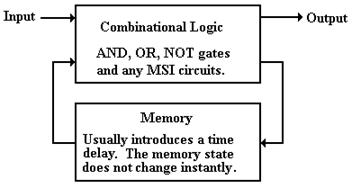The input is fed into the combinational logic (AND gates, OR gates, and NOT gates).  The output of the combinational logic is fed into the memory and available as input to the combinational logic after a specified time delay.

The Idea of a Flip–Flop

Although we have yet to give a formal definition of a flip–flop, we can now give an intuitive one.  A flip–flop is a “bit box”; it stores a single binary bit.  By Q(t), we denote the state of the flip–flop at the present time, or present tick of the clock; either Q(t) = 0 or Q(t) = 1.  The student will note that throughout this textbook we make the assumption that all circuit elements function correctly, so that any binary device is assumed to have only two states.

A flip–flop must have an output; this is called either Q or Q(t).  This output indicates the current state of the flip–flop, and as such is either a binary 0 or a binary 1.  We shall see that, as a result of the way in which they are constructed, all flip–flops also output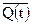, the complement of the current state.  Each flip–flop also has, as input, signals that specify how the next state, Q(t + 1), is to relate to the present state, Q(t).

Every flip–flop also has an input derived from the system clock, which allows it to function as a synchronous circuit.  It also has connections to power and ground.

The Clock

The most fundamental characteristic of synchronous sequential circuits is a system clock.  This is an electronic circuit that produces a repetitive train of logic 1 and logic 0 at a regular rate, called the clock frequency.  Most computer systems have a number of clocks, usually operating at related frequencies; for example – 2 GHz, 1GHz, 500MHz, and 125MHz.  The inverse of the clock frequency is the clock cycle time.  As an example, we consider a clock with a frequency of 2 GHz (2·109 Hertz).  The cycle time is 1.0 / (2·109) seconds, or
0.5·10–9 seconds = 0.500 nanoseconds = 500 picoseconds.

Synchronous sequential circuits are sequential circuits that use a clock input to order events.  Asynchronous sequential circuits do not use a common clock and, as hinted at above, are much harder to design and test.  As we shall focus only on synchronous circuits, we immediately launch a discussion of the clock.

The following figure illustrates some of the terms commonly used for a clock.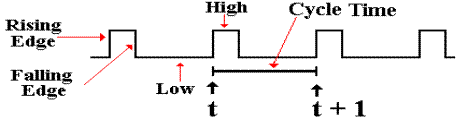The clock input is very important to the concept of a sequential circuit.  At each “tick” of the clock the output of a sequential circuit is determined by its input and by its state.  We now provide a common definition of a “clock tick” – it occurs at the rising edge of each pulse.  We use t to represent the time at a clock tick and (t + 1) to denote the time at the next clock tick – the difference between the two is the clock cycle time.  Suppose a 2 GHz clock, which corresponds to a clock cycle time of 0.5 nanosecond.  Strictly speaking, we should label our timings in nanoseconds: 1.0, 1.5. 2.0. 2.5, etc.  The convention is just to count the ticks, referring to the present clock pulse as occurring at time t and the next one at time (t + 1).

Diversion: What the Clock Signals Really Look Like

The figure above represents the clock as a well-behaved square wave.  This is far from the actual truth, as can be seen by examining the clock pulses with sufficient resolution.  The following figure presents three views of the clock pulse train produced by a typical clock: a realistic physical view and two notations for approximating the clock.

In reality, the clock pulse is not square, but rises and falls exponentially.  For those with mathematical interest, the clock falls in a function of the form e ax and rises with the form of the function 1 – e bx, where a » b.  Use of this precise form does not gain us anything and leads to significant difficulties, so that unless we are troubleshooting at a very low level, we approximate the clock by either a trapezoidal wave or a square wave.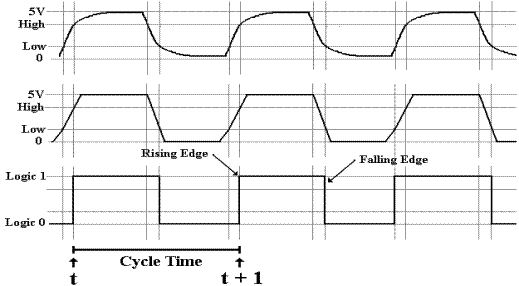The trapezoidal wave form is used when it is important to emphasize the fact that the clock does take some time to rise and fall.  One sees this form of clock representation often when examining timing diagrams for system buses.  The square wave is a further abstraction of the real electrical form of the wave; fortunately it is quite often an adequate representation.  The square wave representation remains at logic 0 until the real electrical clock crosses the threshold for logic high (about 2.5 volts) at which time the square wave jumps to logic 1.  The square wave remains at logic 1 until the real electrical clock signal crosses the threshold for logic low (about 0.8 volts) at which time the square wave goes to logic 0.

In this course, we shall never have to worry ourselves with the actual electrical representation of a clock and seldom shall worry about the trapezoidal representation.  The main point of this diversion is to explain clearly that some logical models are quite useful, even when they do not represent the physical reality with complete accuracy.

The “bottom line” to this argument is that we use the simplest model that will show what we need to see in considering a problem.  Here, we find that the only real requirement for a clock is to differentiate the “present state” from the “next state”; a square wave clock will do.

The NOR Gate and an SR Latch

We now begin our investigation of flip–flops. We begin with the SR flip–flop, which is the simplest.  In order to understand an SR flip–flop, we must first discuss the SR latch.  In order to understand the SR latch, we must review the properties of the NOR gate.

The NOR gate, as depicted in the following diagram, is logically equivalent to an OR gate followed by a NOT gate.  In reality, it is faster than its equivalent; a typical NOR has a gate delay of 10 nanoseconds; the gate delays total 23.5 nanoseconds for the OR/NOT circuit.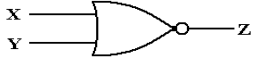As we have seen previously, the truth table for the NOR gate can be written as follows.

 Y X Z 0 0 1 0 1 0 1 0 0 1 1 0

To support our next discussion, it will prove useful to reduce this table to two equations.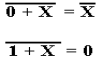Given these equations, we consider the following circuit with cross–coupled NOR gates.  We stipulate that the two inputs to the circuit are both held at logical zero.  At this point, we do not know the value of Q or care about it; just that the two outputs are complements.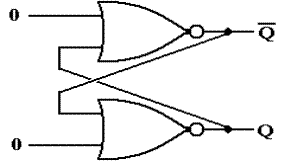Rewriting the top equation with X first as Q and then with X as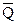, we have these equations.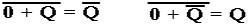An examination of this circuit shows it to be stable.  The top NOR gate has as input 0 and Q; its output is.  The bottom NOR gate has as input 0 and; its output is Q.  The output of each gate is precisely the previous input to the other gate, so nothing changes.

As we shall see, things become interesting when one of the inputs becomes 1.

We now imagine the circuit in one of two logically consistent states (where Q andare not equal) and change the top input to logic 1.  At first, the situation is little changed, as the NOR gates have not yet reacted to the new input.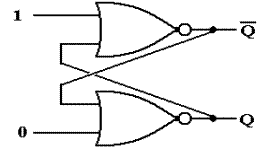Note that the input to the top device is now 1 and Q (which is either 0 or 1).  The inputs to the bottom NOR gate have yet to change and will not until after one gate delay.  Recall that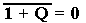for each of the two possible values of Q.  After one gate delay, the output of the top NOR gate has changed to 0, but that the output of the bottom NOR gate has yet to respond to its new input, which has just arrived.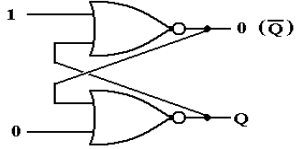After the second gate delay, the output of the bottom NOR gate becomes 1, as
NOR(0, 0) = 1.  The input to the top NOR gate might change, but its output will not change, as NOR(1, 1) = 0.  The circuit is now stable.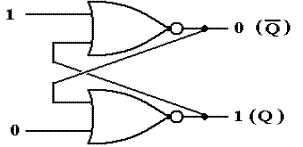Let’s review what happened and clarify some of the labeling.  At the beginning, the device had some state, denoted by Q in the diagram.  It will be called Q(t) when we have a clocked device, but for now it is just “Q”.  Changing the value of the top input to 1 and leaving the bottom input at 0 induces some changes that take two gate delays to complete.  The circuit is now stable, with a value of Q = 1.  This is the “new value of Q” as opposed to the “old value of Q”, which might have been 1, but just as easily could have been 0.

We now go back to the circuit in one of two logically consistent states (where Q andare not equal) and change the bottom input to logic 1, leaving the top input as 0.  At first, the situation is little changed, as the NOR gates have not yet reacted to the new input.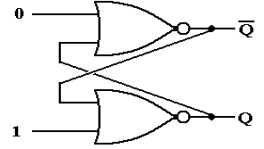Note that the input to the bottom device is now 1 and(which is either 0 or 1).  The inputs to the bottom NOR gate have yet to change and will not until after one gate delay.  Now: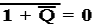for each of the two possible values of.  After one gate delay, the output of the bottom
NOR gate has changed to 0, but that the output of the top NOR gate has yet to respond to its new input, which has just arrived.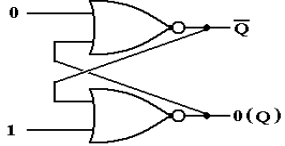After the second gate delay, the output of the top NOR gate becomes 1, as NOR(0, 0) = 1.  The input to the bottom NOR gate might change, but its output will not change, as
NOR(1, 1) = 0.  The circuit is now stable.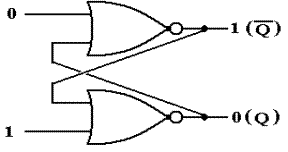We note in passing the fourth possible input combination.  Recall that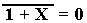for any possible value of X.  Suppose that both the top and bottom inputs are set to 1.  The only possible outputs are Q = 0 and= 0.  This is obviously invalid.

What we have here is called an SR Latch.  The name SR comes from “Set Reset”.  The general diagram for the SR latch is as below, where the S input is the “Set input” and the R input is the “Reset input”.  When a latch is set, its value becomes 1.  When a latch is reset, its value becomes 0.  In this figure, the value is denoted by Q and its complement by.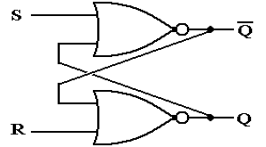Supposing that the old value of the SR latch is denoted by Q, we have the following truth table to describe the response to the input.  Such a table is called a “characteristic table”.

 S R New Value 0 0 Q 0 1 0 1 0 1 1 1 Invalid

The Clocked SR Latch
The circuit above is interesting (at least to the author of this textbook), but somewhat troublesome.  The problem is that it will change state any time the input changes.  Normal design practice, especially in synchronous circuits, demands that the change take place only during certain phases of the system clock.  The circuit to accommodate this design constraint is called either a “clocked SR latch” or a “level triggered SR flip–flop”.  The circuit diagram for a clocked SR latch is shown in the following figure.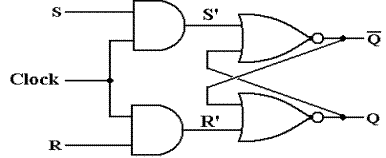This circuit is just an SR latch with the input passed through a pair of AND gates.  When the input Clock = 0, each of S’ and R’ are 0 without regard to the values of S and R.  The latch does not change state, but keeps its current value.  When the input Clock (also called “Enable”, “Strobe”, or just “Wake Up and Look at the Input”) is 1, the SR latch responds to the input values of S and R as discussed above.

The Clocked D Latch
The clocked D latch is a specialization of the clocked SR latch.  The term “D” in “D Latch” and later in “D Flip–Flop” indicates “Data” and shows that the device stores data.  When the clock input is 1, the device will store its input.  The D latch can be constructed from an SR latch as indicated in the following circuit diagram.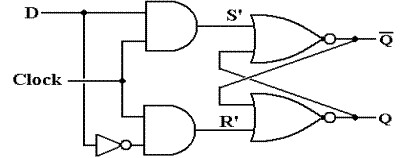Note that when Clock = 0, both S’ = 0 and R’ = 0, without regard to the value of D.  When Clock = 1, then each of S’ and R’ depend on D; S’ = D and R’ =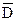.  This leads to the following characteristic table for the latch.

 Clock D S’ R’ New Value 0 0 0 0 Q 0 1 0 0 Q 1 0 0 1 0 1 1 1 0 1

In a clocked latch, the ability to respond to input is connected to an external signal, called “Clock”.  This signal is based on the system clock, but might be modified as needed.  The next figure shows a circuit that loads dependent on a signal called “Load”.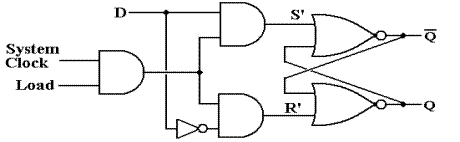The signal called “Load” is obviously generated by a control circuit that is synchronous with the system clock.  The timing diagram for this signal might be as follows.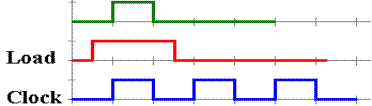The SR latch accepts input if and only if both Clock = 1 and Load = 1.

Notation for Latches and Flip–Flops
When showing either latches or flip–flops as circuit elements, it is undesirable to show the “internals” of the device.  We need a simple schematic for latches and flip–flops that shows what we need to see and no more.  Here is the standard symbology for each of SR and D circuits, both flip–flops and latches.

The notation adopted is a simple box notation, with the outputs and inputs shown.  The difference between the symbol for a latch and that for a flip–flop lies in the handling of the clock input.  A flip–flop, also called an “edge triggered flip–flop” is shown with a small triangle attached to the clock input.  For SR and D devices, we have the following symbols.

Here are the symbols for SR and D latches.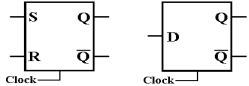Here are the symbols for SR and D flip–flops, which we have not yet defined.
Note the small triangles on the clock input; this says “edge triggered”.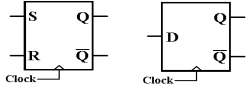One should note that the more common symbols for flip–flops show the clock input coming
in “from the side” as shown in the next figure.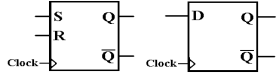Clocked Latches and Flip–Flops
The two most common synchronous sequential devices are clocked latches and flip–flops.  In a few pages, we shall see that a flip–flop is essentially a clocked latch that has been modified.  For the moment, we shall just note that both clocked latches and flip–flops change state in response to the input, sampled at a fixed part of the system clock cycle.

The standard way to describe each of these devices uses a characteristic table, which defines the state at the next clock tick in terms of the input and the state at the present clock tick.  Recalling our notation that “t” stands for the current clock tick and “t + 1” stands for the next clock tick, we say that we determine Q(t + 1) in terms of Q(t) and the input.

One should note that clocked latches and flip–flops share characteristic tables, so that the characteristic table for an SR latch would also be that for an SR flip–flop and the characteristic table for a D latch would also describe a D flip–flop.  The difference between clocked latches and flip–flops lies in how the device reacts to the clock.

The Feedback Problem with Clocked Latches.
The problem with clocked latches is that they respond to the input whenever their clock input is set at logic one.  For the above example, this occurs whenever both the System Clock and the Load signal are set to logic 1.  As good design practice calls for Load to go to logic 1 before the rising edge of the system clock and remain at 1 past the falling edge, the latch will respond to input during a time interval equal to at least one half of the clock cycle time.

Consider a circuit in which there is feedback to the circuit.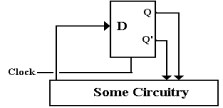As a concrete example of the problem, we consider an otherwise silly circuit that has the potential for displaying the timing issues very clearly.  In this circuit, we have D =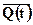, with the intention of having Q(t + 1) =; the circuit oscillates.  As with any sufficiently simple example, there are obvious ways to get this done more easily; that is not the point.  The circuit that we consider is shown below.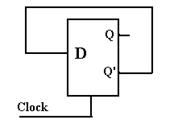The key issue here is the propagation delay for the line feeding the D input; the interval between the time that Q’ changes and the time at which D changes.  Were that interval greater than one half of the system clock period, the circuit might function as expected.

Here is the timing diagram for what we intend to happen.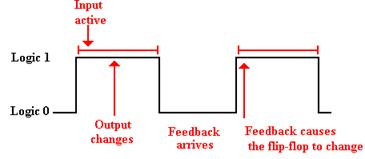Note that the feedback cannot loop back to the D input either too early or too late.  It is rarely the case that the input arrives too late; this is a problem that is easy to solve.  However, it is very likely that the feedback will arrive too early.  This will cause instabilities.

Consider what might happen when the interval for propagation is much less than the clock period.  In this next example, we make the reasonable assumption that the D input changes 0.5 gate delays (for the clocked D latch) after the output Q changes, and that the clock period is somewhat greater than 10 gate delays.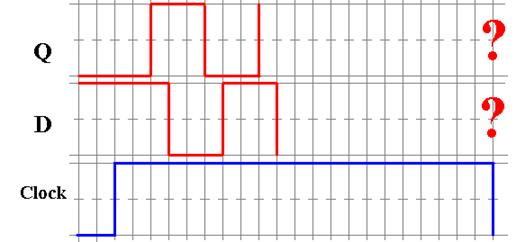At the beginning of this time trace, we have a consistent situation.  Q = 0 and D == 1.  The clock then goes high and stays high for a number of gate delays.  We have the following sequence of events, with the times measured in multiple of gate delays.

T = 0.0          The clock goes high and the D latch responds to its input.
T = 1.0          The value of Q changes in response to the new value of D.
T = 1.5          The value of D changes, as it is equal to.
T = 2.5          The value of Q changes again.
T = 3.0          The value of D changes again
T = ??           The clock goes low and the value of Q is unpredictable.

Please note that the unpredictability of the output of the flip–flop is due to the uncertain relation between the clock time and the sum of the gate delay times for the flip–flop.  In general, the clock cycle time will be known to a very high accuracy (1 part in a million, or better), but the gate delay times are only approximations, quoted as averages.

The problem lies in the fact that, even with the use of a clocked latch, we have lost control of the feedback loop.  This has lead to instability.  We now consider the first of two possible solutions to managing the feedback loop; this is called a “Master/Slave” flip–flop.

We have two problems with the nomenclature.  The first is that the device is not a flip–flop in the current sense of the word.  It is just a clocked latch that is called a flip–flop.

The second issue we have with the nomenclature is the term “Master/Slave”.  To be blunt, the term is not politically correct and we do like to be politically correct whenever possible.  Some time ago, the IEEE (Institute of Electronic and Electrical Engineers) sponsored a contest through its magazine “Computer” to find an alternative name for this device.  All reasonable answers were published in the journal; all were ridiculous and some quite suggestive of practices best left unmentioned in polite company.  The bottom line is that we are stuck with the term if we discuss latches; with real flip–flops the problem goes away.

The D Master–Slave Latch
Basically, a Master–Slave device is fabricated from two clocked latches by joining them, as follows.  Note that the second latch is often an SR latch, as it is simpler.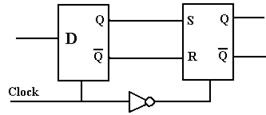Here we note that a correctly functioning D latch will never allow S = 1 and R = 1 to be input to the second latch, so the SR undefined input does not arise.  The important feature of this arrangement is the NOT gate in the clock line.  When the D latch accepts input, the SR latch cannot; and when the SR latch accepts input, the D latch cannot.

To see the functioning of this combination, let us again examine the above silly circuit, this time implemented with a Master/Slave D latch.  First we see the circuit, then the timings.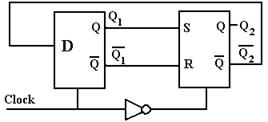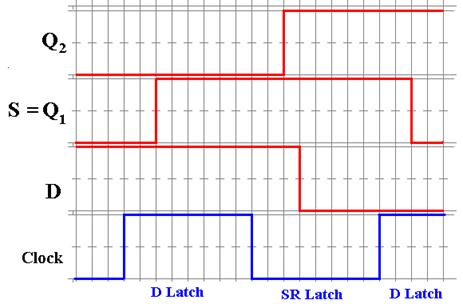At the start, we have Q2 = 0 and D = 1, with the D latch not sensitive to its input.  When the clock becomes high, the D latch becomes sensitive to its input, and Q1 changes after a time to allow for the gate delays in the latch.  Now the SR latch has S = 1 and R = 0 (not shown), but it is not yet sensitive to these inputs.  When the clock goes low, the SR latch accepts its input and after its gate delays changes its output.  Almost immediately, the value of D changes, but the D latch is no longer sensitive to its input.  The D input takes effect at the next clock pulse.

What the Master/Slave latch accomplishes is to control the feedback loop by causing the output to be delayed until the time at which the master latch is no longer sensitive to its input.  When the clock next goes high, the master latch responds and a new cycle begins.

Level Triggering and Edge Triggering

The feedback problem that was addressed by the Master/Slave latch was due to the fact that the master latch was sensitive to its input for a full half of the clock cycle.  We now examine another solution to this problem, one that makes the latch sensitive for a much shorter time.  We begin by reviewing the concept of level triggering, then introduce the idea called “edge triggering”, and conclude by designing a mechanism that allows for edge triggering.

To review, a clocked latch is sensitive to its input during one half of the clock cycle, usually when the clock is “high” (at logic level 1), but not necessarily so.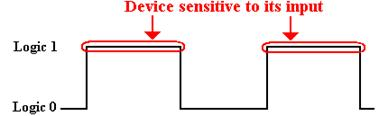By definition, an edge triggered flip–flop is sensitive only during an edge of the clock pulse.  We can have positive edge triggering, as shown just below.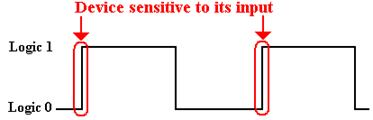We can also have negative edge triggering, as shown just below.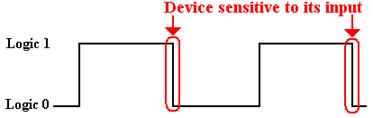By way of giving the precise definition of a flip–flop, we quote Andrew S. Tanenbaum.

For emphasis, we will repeat the difference between a flip–flop and a latch.
A flip–flop is edge triggered, whereas a latch is level triggered.  Be warned, however, that in the literature these terms are often confused.  Many authors
use “flip–flop” when they are referring to a latch, and vice versa.

(     Andrew S. Tanenbaum, Structured Computer Organization, Fifth Edition,
Pearson/Prentice–Hall, 2006.  ISBN 0 – 13 – 148521 – 0.  Page 162 )

Flip–Flops
By definition, a flip–flop is an edge–triggered latch.  We must now show how to achieve edge triggering.  In essence, what we shall do is take a level triggered latch and give it a clock pulse with a very short positive (logical 1) phase.

The key component of an edge–triggered flip–flop is a pulse generator that we studied in a previous chapter.  This could be based on the gate delay of a NOT gate.  More modern devices likely use a different circuit so as to generate a shorter pulse.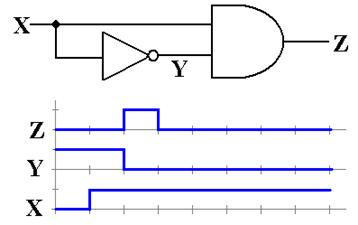Now that we have a method to generate a short pulse, we can build an edge–triggered device.  The following is a diagram of the components of two typical edge–triggered flip–flops.  The top one is a SR flip–flop and the bottom one is a D flip–flop.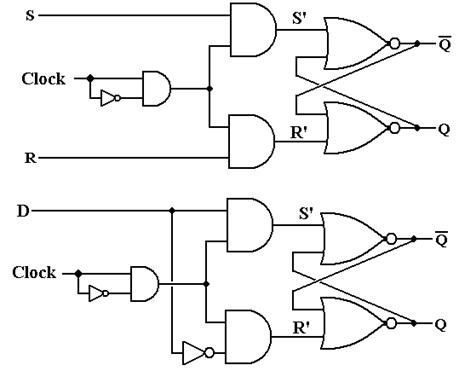Feedback for Flip–Flops
Part of our motivation for master/slave latches, discussed above, was the problem of feedback.  We now pose the same problem in our consideration of flip–flops.  In order to avoid the simple and silly example used the first time, we shall examine the general case.

Here is the circuit for consideration.  We postulate a total of gate delays (including that of the D flip–flop) to be the time interval signified by D.  Thus at time D after the D latch is first sensitive to its input, the circuitry has output a new value to become D.  But the flip–flop is activated by a short pulse, of  time length d.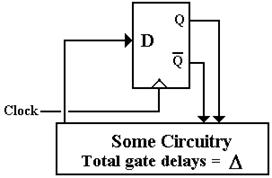The timing diagram below shows the interruption of the uncontrolled feedback loop.  By the time that the output of the circuitry has changed, the D flip–flop is no longer sensitive to input.  The input will not become effective until the beginning of the next clock cycle.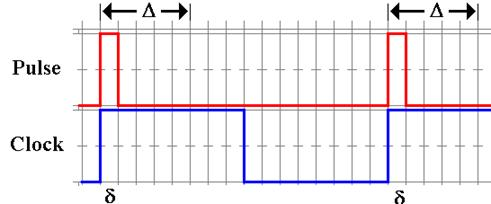How can one insure that the relative timings of two circuits, the D flip–flop and the rest of the CPU circuitry, operate with the correct timings?  This is one of the issues of central importance in the design of a CPU; since we have stumbled into it, let’s talk about it.

To be more precise, define two total gate delays: DMIN and DMAX.  DMIN is the total time delay for the fastest CPU operation and DMAX. the delay for the slowest.  We must have
DMIN > d, or the circuit would occasionally display uncontrolled feedback.  Conservatively, we might say DMIN ³ 1.5·d.  The next criterion is a bit more difficult to state precisely, but it might be stated something like T ³ 1.5·DMAX, where T denotes the clock period.  What we say here is that the clock period must be long enough for the CPU output to “settle”.  Note, however, that a value of T much larger than DMAX is just wasted time.

Put another way, the value of DMAX determines the fastest clock that can reasonably be applied to the CPU.  A good part of the art of CPU design is based on this issue.  When we study the RISC (Reduced Instruction Set Computer) movement, we shall see that one of the issues was to remove the more complex instructions, thus reducing DMAX and allowing for a faster clock.  There is a trade–off here that we shall explore in later chapters.

To be honest, the sum of CPU gate delays is not always the limiting factor in the clock speed.  Some recent CPUs have been designed with a “hot clock” that is hot in both ways.  It is very fast, being of the order of 4 to 5 GHz.  It is also hot in the literal sense, in that the CPU, operating at that clock rate, emits so much heat that it overheats itself.  The art of CPU design is always bumping up against those messy laws of physics.

The SR Flip–Flop
We have completely defined the SR flip–flop, based on the idea of an SR latch.  While we could just leave the topic and proceed, it is appropriate at this time to review the subject and state, in one place, what the student is expected to know.  We begin with a depiction of the SR flip–flop that will be used in all future discussions.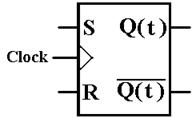At this point we are no longer interested in the internal construction of the flip–flop, but on its operational characteristics.  These are given in the characteristic table.

 S R Q(t + 1) 0 0 Q(t) 0 1 0 1 0 1 1 1 ERROR

We next address an issue that commonly arises in the use of flip–flops in circuit design.  We have a number of scenarios.  For each, we know Q(t) and what Q(t + 1) should be. The question is how to achieve that change.  For example, if Q(t) = 0 and we want Q(t + 1) = 0, we have two choices: either S = 0 and R = 0, or S = 0 and R = 1.  The first option, keeps the state unchanged at 0; the second forces it to 0.  As S = 0 is sufficient to do this without regard to the value of R, we say that the input is S = 0 and R = d; the d standing for “don’t care”.

On the other hand, if Q(t) = 0 and Q(t + 1) = 1, only S = 1 and R = 0 will do.  This is the only combination that will give a next state of 1 when the present state is 0.

If we have Q(t) = 1 and want Q(t + 1) = 0, then our choice is simple: S = 0 and R = 1.

If we have Q(t) = 1 and want Q(t + 1) = 1, then we can choose either S = 0 and R = 0, or
S = 1 and R = 0.  This is denoted as S = d and R = 0.

The above discussions lead to the excitation table for the SR flip–flop.

 Q(t) Q(t + 1) S R 0 0 0 d 0 1 1 0 1 0 0 1 1 1 d 0

The JK Flip–Flop: Enhancing the SR Flip–Flop
Recall the characteristic table of the SR flip–flop.  We repeat the table here for emphasis.

 S R Q(t + 1) 0 0 Q(t) 0 1 0 1 0 1 1 1 ERROR

The theoretician examining this table would note two facts immediately.
1.   The input S = 1 and R = 1 is disallowed; we would like to do something with it.
2.   The values for Q(t + 1) are three of the possible four Boolean functions of 1 variable.

Considering Q as a Boolean variable, we now show that there are exactly four Boolean functions of this Boolean variable.  These are f(Q) = 0, f(Q) = 1, f(Q) = Q, and f(Q) =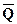.  We do this by showing the truth table for each of these functions and noting that there are only four different ways to put 0’s and 1’s into the two row entries for a function..

 Q 0 Q1 0 0 0 1 1 1 0 1 0 1

Table: The Four Boolean Functions of Boolean Variable Q

Given this, our enhanced SR flip–flop would have Q(t + 1) =(t) as one possible output.  For maximal compatibility with the existing SR, we would want to leave the existing valid inputs alone and just make good use of the invalid one.  What we get is a JK flip–flop.

Recalling that an SR flip–flop is so called because it is a Set–Reset device, we may ask for the meaning of JK.  One is tempted to make up some story related to names in German, but the basic answer is that “I don’t know”.  In any case, we present the characteristic table for the JK flip–flop and then ask how one might modify an SR flip to achieve that goal.

Here is the desired characteristic table.

 J K Q(t + 1) 0 0 Q(t) 0 1 0 1 0 1 1 1(t)

Viewing this as a modification of the SR flip–flop, we ask how to generate each of S and R from the inputs J and K under the following constraints;
1.   Except when J = 1 and K = 1, we want to have S = J and R = K.
Under these circumstances, the behavior is identical.

2.   When J = 1, K = 1, and Q = 0, we want S = 1 and R = 0.  This makes Q(t + 1) = 1.

3.   When J = 1, K = 1, and Q = 1, we want S = 0 and R = 1.  This makes Q(t + 1) = 0.

When in doubt about how to create a circuit, we make a truth table.  The inputs to the truth table are J, K, and Q (the present state).  The outputs are S and R.

 Row Q J K S R 0 0 0 0 0 0 1 0 0 1 0 1 2 0 1 0 1 0 3 0 1 1 1 0 4 1 0 0 0 0 5 1 0 1 0 1 6 1 1 0 1 0 7 1 1 1 0 1

We have two patterns that almost work: S = J·and R = K·Q.  Let’s examine each case.

S = J·produces the expected result for all rows except row 6.  In that row we have
Q = 1, J = 1, and K = 0.  We want Q(t + 1) to be 1.  But S = 0 and R = 0 will cause
Q(t + 1) = Q(t) = 1, exactly what we want.  So this simple formula causes no trouble.

R = K·Q produces the expected result for all rows except row 1.  In that row we have
Q = 0, J = 0, and K = 1.  We want Q(t + 1) to be 0.  But S = 0 and R = 0 will cause
Q(t + 1) = Q(t) = 0, exactly what we want.  So again we have no trouble.

Here is the basic detailed circuit for the JK flip–flop.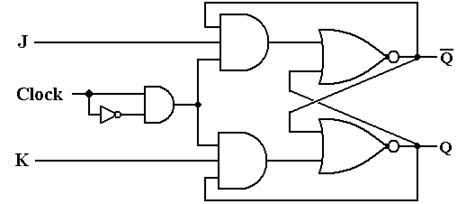We now give the standard representation of the JK flip–flop as will be used in future discussions.  Again, note the triangle symbol on the clock input, indicating edge triggering.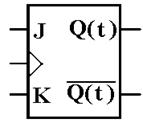We have already presented the characteristic table for the JK flip–flop.  Here it is again.

 J K Q(t + 1) 0 0 Q(t) 0 1 0 1 0 1 1 1(t)

We now create the excitation table for the JK.

If Q(t) = 0 and Q(t + 1) is to be 0, we can use either J = 0 and K = 0, or J = 0 and K = 1.

If Q(t) = 0 and Q(t + 1) is to be 1, we can use either J = 1 and K = 0, or J = 1 and K = 1.
Note that this is a new option, not available for an SR flip–flop.

If Q(t) = 1 and Q(t + 1) is to be 0, we can use either J = 0 and K = 1, or J = 1 and K = 1.
Note that this also is a new option, not available for an SR flip–flop.

If Q(t) = 1 and Q(t + 1) is to be 1, we can use either J = 0 and K = 0, or J = 1 and K = 0.

This gives the excitation table for the JK flip–flop.

 Q(t) Q(t + 1) J K 0 0 0 d 0 1 1 d 1 0 d 1 1 1 d 0

The D Flip–Flop
We have already examined the D latch.  The input is labeled “D” for “Data”.  The D flip–flop has a characteristic table identical to that of a D latch.

 D Q(t + 1) 0 0 1 1

The device is so simple that it does not require an excitation equation.  We just use an excitation equation, which simply states “Give it what you want”.

D = Q(t + 1)

The T Flip–Flop
This is the fourth and last of the major types of flip–flops.  The input to this flip–flop is labeled “T” for toggle.  When T = 0, the state remains the same.  When T = 1, the flip–flop changes state.  This gives rise to the following characteristic table.

 T Q(t + 1) 0 Q(t) 1(t)

The excitation table for this flip–flop is almost obvious.

 Q(t) Q(t + 1) T 0 0 0 0 1 1 1 0 1 1 1 0

This gives rise to an excitation equation.

T = Q(t) Å Q(t + 1)

The JK As a General Flip–Flop
We now take notice that the JK can be configured to function as any of the other 3 flip–flop types.  In this, it is the most general type of flip–flop.

The JK As a D Flip–Flop
To convert a JK to a D flip–flop, connect the inputs as follows.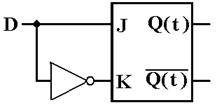If D = 0, then J = 0, K = 1, and Q(t + 1) = 0,  If D = 1, then J = 1, K = 0, and Q(t + 1) = 1.

The JK As A T Flip–Flop
To convert a JK to a T flip–flop, connect the inputs as follows.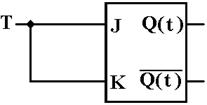If T = 0, then J = 0, K = 0, and Q(t + 1) = Q(t).
If T = 1, then J = 1, K = 1, and Q(t + 1) =(t)

## Registers

A register is a storage device capable of holding binary data.  It is best viewed as a collection of flip-flops, usually D flip-flops.  To store N bits, a register must have N flip-flops, one for each bit to be stored.  We show a design for a four-bit register with a synchronous LOAD.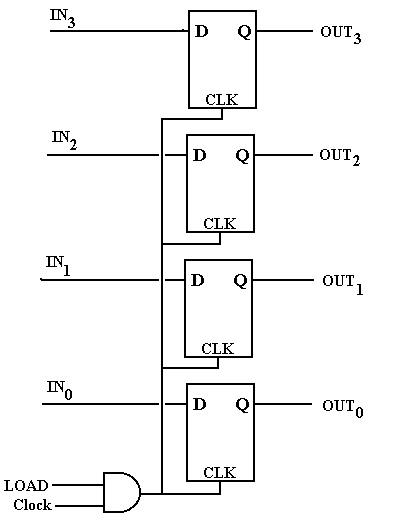In this example, the 4-bit register is implemented by four D flip-flops.

Note the input CLK comes from an AND gate that puts out the logical AND of the system clock (Clock) and the LOAD signal.  When LOAD is 0, the flip-flops are cut off from the input and do not change state in response to the input.  The design calls for LOAD to be 1 for almost one clock pulse, so that the system clock and LOAD are both high for 1/2 clock cycle.  At this time, the register is loaded.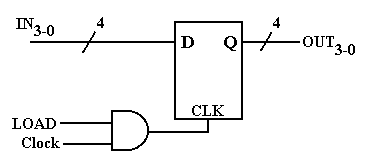The figure at right shows a short-hand notation used when drawing registers that contain a number of flip-flops identically configured.

It should be obvious that the figure represents a 4-bit register.

Definition:  A register file is a collection of registers that is considered logically as a unit, and each of which can be individually addressed.  For example, one modern computer has thirty–two general purpose registers in the CPU, denoted in its assembly language as
%R0 through %R31.  This collection of registers is considered a register file.  Admittedly, the terminology is rather old, and might be obsolete, but it works for this author.

Consider a register file containing two 4-bit registers.  The registers will be named R0 and R1, and selected by a one-bit control signal, called R; R = 0 selects R0 and R = 1 selects R1.  Note that the figure was drawn before this author changed the notation to %R0 and %R1.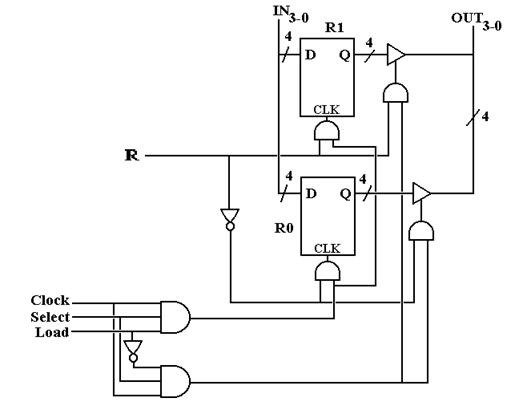Analysis of this diagram shows how it works.  Begin with the interaction of the Load and Select signal.  If Select = 0, neither register will be active.  If Select = 1, the selected register will be loaded if Load = 1 and read (contents copied out) if Load = 0.

There may be other options for controlling such a collection of registers, but all such require two control signals as there are three options for a register
1)   Do nothing – save the current contents
2)   Copy the input to the register and possibly change its contents
3)   Copy the register contents to the output.  Also save the contents.

We now present a register file structure that will be of importance in our design of a model computer.  To conform to the design used later in the book, this figure should show eight
32–bit registers for a total of 224 flip-flops (8 · 32 = 256 and 7 · 32 = 224, we explain this apparent contradiction a bit later), which would present difficulties in drawing.  For the sake of simplicity and legibility, we present a file of four two–bit registers, with a two-bit input bus on the left and a two-bit output bus on the right.
The signal Bus ® Reg causes the selected register to be loaded from the bus at left.
The signal Reg ® Bus causes the contents of the selected register to be copied out.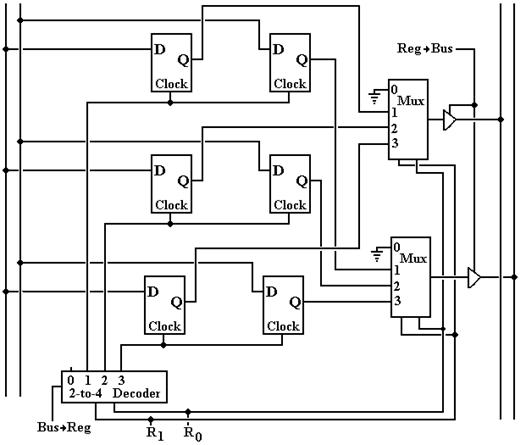Something Is Missing

Recall that an N–bit register requires N flip–flops; hence a two-bit register requires two
flip-flops and a register file of four two–bit registers requires eight flip-flops.  Yet our design above has only six flip-flops.  The observant student will notice that there seems to be no register %R0.  We seem to have three fully functioning two-bit registers that we could name as %R1, %R2, and %R3.

To understand the functioning of the register file above, let us focus on a single bit and both its flip-flops and circuitry for reading and writing those flip-flops.  We first consider the output circuitry, controlled by a 4-to-1 multiplexer and an enabled-high tri-state buffer.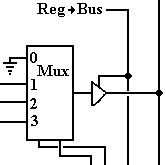The two bits entering the multiplexer from the bottom are the control bits for the multiplexer and represent the 2-bit binary code for the register to be selected.  If the signal Reg ® Bus is active, then the output of the multiplexer is placed on the output bus.  It is easy to see that if the binary codes are 01, 10, or 11 that the contents of the flip-flop for %R1, %R2, or %R3 are output to the bus.  But if the code is 00, the output bus is connected to ground, effectively placing a 0 on the output bus.

We now consider the input circuitry.  Note that the inputs of every flip-flop associated with this bit are directly connected to the appropriate bit-line of the input bus and that the register to receive the input is activated by its CLOCK input.  Here is the circuitry for producing the CLOCK input to the flip-flops associated with the register that is selected for input.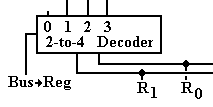If the signal Bus ® Reg is not active, the decoder is not enabled and all of its output lines are inactive.  Thus, no register is selected for input.  If Bus ® Reg = 1, then the flip-flops for that register receive a CLOCK pulse and accept the input from the appropriate bit-line of the input bus.  This works well for register codes 01, 10, and 11.

But what about register-select code 00?  If R1R0 = 00 and Bus ® Reg = 1, output 0 of the decoder is active but is not connected to anything.  To be blunt, no register is loaded.

The Phantom Register %R0

Let us review what happens when the register select codes are R1R0 = 00, indicating a selection of register %R0.
If Reg ® Bus is asserted, the number 0 is copied out to the output bus.
If Bus ® Reg is asserted and Reg ® Bus is not asserted, nothing happens.

What we have here is (%R0) º 0, that is register 0 is a constant register holding the constant value 0.  Registers that hold constant values are not implemented with flip-flops, but with connections to ground (logic 0) and to voltage (logic 1).  The use of a register with its select code equal to decimal 0 greatly facilitates the design of the control unit of the CPU.

As we design the control unit, we shall add other constant registers that are not part of the general purpose register set.  Unlike %R0, these will not be accessed by the assembly language, but are used by the control unit.  The most likely candidate is the constant 1, depicted in binary as 0000 0000 0000 0000 0000 0000 0001 – bit 0 is connected to positive voltage (logic 1) and bits 1 through 31 connected to ground (logic 0).

Why Have a Register for Zero?

The above discussion suggests a valid question with an entirely valid answer.  To jump ahead, we postulate a three-bus design with the buses labeled as B1, B2, and B3.  Suppose I want to transfer the contents of B1 to B3, in RTL (Register Transfer Language) B3 ¬ (B1).  One way would be to set B2 to all zeroes and have B3 ¬ (B1) + (B2); thus implementing the algebraic equation Y = X + 0 rather than the equivalent Y = X.  The reason that we must set the contents of B2 to 0 in order for this to work is that otherwise the contents of B2 would be undefined and might take any possible value as dictated by electrical noise.

As we develop the logic of the control unit for the CPU, we shall see why it is simpler to issue the control signal B3 ¬ (B1) + (B2) with (B2) º 0, rather than the apparently simpler control signal B3 ¬ (B1).  It all comes down to making a simpler and faster CPU.

More on Register Files

We now consider the idea of a register file as used later in the design of our model CPU.
This is a collection of eight registers, labeled %R0 through %R7, each a 32-bit register.
As 8 = 23 registers, there are three register select lines, labeled R2R1R0.

Our CPU design will call for three CPU buses, labeled B1, B2, and B3.  Buses B1 and B2 will be used as input to the ALU (Arithmetic-Logic Unit, the part that does the math), and bus B3 will be used as output of the ALU.  Thus the register file will support output to two buses (B1 and B2) and input from a single bus (B3).

The register file for this design has
32 input lines, labeled IN31 - 0

Two sets of32 output lines,  each labeled OUT31 - 0

3 register select lines, labeled R2 – 0 or alternatively R2R1R0.

Reg ® B1, Reg ® B2, B3 ® Reg, and Clock signalsFigure: The Register File for Our Computer and Its Data Buses

## Registers: Some Miscellaneous Remarks

We now consider a few designs for registers to be constructed from flip-flops.  Since the issues to be considered do not depend on the number of bits to be stored in the register, we consider a 1–bit register.  Consider the following design.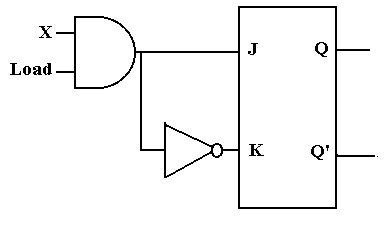The input to this circuit is X.  When Load = 1, the output of the AND gate is X and the input to the flip-flop is J = X and K = X’, as desired.

The problem arises when the register is to keep its contents and Load = 0.  The output of the AND gate is 0 and the input to the flip-flop is J = 0 and K = 1.  The flip-flop is cleared; the auto-forget option.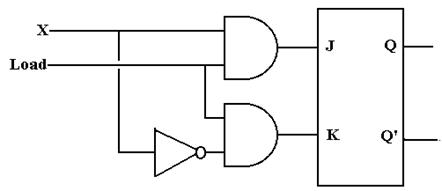The correct circuit is shown at the right.  When Load = 1, the input to the flip-flop is J = X and K = X’, as desired.

When Load = 0, the input to the flip-flop is J = 0 and K = 0, and the flip-flop maintains its current state.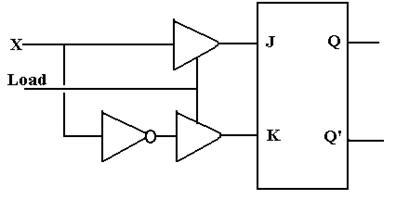We now consider the use of tri-state buffers to control input.  When Load = 1, the circuit operates as desired.  But consider Load = 0.  Both tri-states are disabled, giving a high-impedance output, which is interpreted as logic 1 by the flip-flop.  When Load = 0, we have J = 1 and K = 1 and that is trouble.

The bottom line: tri-states cannot be used on input.

## Shift Registers

We now consider the following circuit built from four D flip-flops.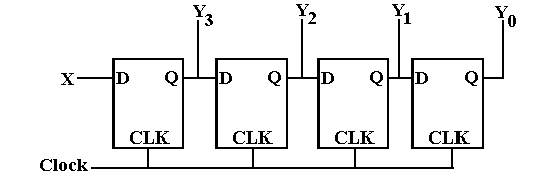Before each clock pulse, D3 = X and DK = QK+1 for 0 £ K £ 2.  If QK(T) is the state before the clock pulse and QK(T + 1) is the state after the clock pulse, then the situation after the clock pulse is given by Q3(T + 1) = X and QK(T + 1) = QK+1(T) for 0 £ K £ 2.  The input bits in X are thus seen to be shifted across the four flip-flops, hence the name.

Since we don’t like to draw too many flip-flops, we have a symbol for shift registers.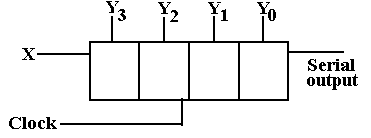The shift register has both serial

input and parallel input.  The

parallel input is through the lines

labeled by the Y’s, a notation we

have normally used for output

only.  Two common modes are

parallel in / serial out and

serial in / parallel out.

Shift registers are of great use for handling serial Input/Output devices, which transmit data one bit at a time.  Consider the case of a character ‘B’ coming in on a serial input line.  The ASCII character code for ‘B’ is 42 hexadecimal, so the binary input is 01000010.  This input is passed into an 8-bit shift register.  After 8 clock cycles, the state of the shift register is
Y7 = 0, Y6 = 1, Y5 = 0, Y4 = 0, Y3 = 0, Y2 = 0, Y1 = 1, and Y0 = 0.  This is then transferred into the CPU as parallel data.  For output, the character code is transferred in parallel into the shift register and then shifted out one bit at a time, producing the serial bit stream.

More detailed study of serial I/O indicates that there are a few more details to be considered.

We now consider schemes for data transfer between shift registers.  We first consider in detail a design shown in some textbooks.  We then show a preferred design.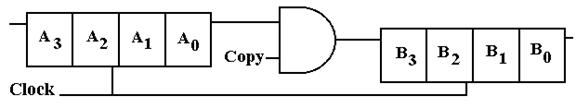This circuit, copied from another textbook, has many problems.  A complete analysis of the circuit depends on the input to the flip-flop labeled A3.  If the input is undefined, it will be interpreted as a 1.  If it is logic 0, then zeroes will be shifted in.  For our analysis, we shall assume that the input is specified by a proper source, thus avoiding these problems.

Suppose that the Copy control signal goes to 1 at T = 0, when the contents of registers A and B are X3X2X1X0 and Y3Y2Y1Y0, respectively.  We analyze this circuit and find it has many problems.  The A register is cleared rather than copied.

 Time Copy A3 A2 A1 A0 B3 B2 B1 B0 0 1 X3 X2 X1 X0 Y3 Y2 Y1 Y0 1 1 0 X3 X2 X1 X0 Y3 Y2 Y1 2 1 0 0 X3 X2 X1 X0 Y3 Y2 3 1 0 0 0 X3 X2 X1 X0 Y3 4 0 0 0 0 0 X3 X2 X1 X0 5 0 0 0 0 0 0 X3 X2 X1 6 0 0 0 0 0 0 0 X3 X2 7 0 0 0 0 0 0 0 0 X3 8 0 0 0 0 0 0 0 0 0

The problem with this circuit is that the system clock is sent directly into the clock input of the shift registers, so they shift continuously.  The input to A (presumed 0) is shifted first into A and then into B.  At the end, all information is lost.

The correct shift register uses the Copy control signal to pass the clock signal to the shift registers or keep it from getting to them.  It also re-circulates the A register to prevent loss.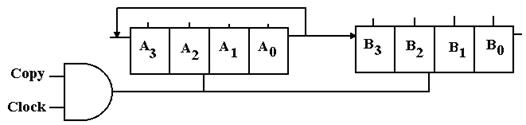Solved Problems

PROBLEM

1.   A Set-Dominate flip-flop acts like the SR flip-flop except that S = R = 1 sets the flip-flop.
Show the characteristic table and the state table for a set-dominate SR flip-flop.

The state table for the flip-flop may be written as follows.

 S R Q(T+1) Comments 0 0 Q(T) Same as SR 0 1 0 Same as SR 1 0 1 Same as SR 1 1 1 This is the only difference.

There is another form of the state table that is occasionally used.  The instructor prefers state tables in the form above, but presents an equivalent state table below.  Those students who prefer the form of the following table should feel free to use it.

 PS \ Input S = 0, R = 0 S = 0, R = 1 S = 1, R = 0 S = 1, R = 1 Q(T) = 0 0 0 1 1 Q(T) = 1 1 0 1 1

Again, note that only in column for S = 1 and R = 1 do we see a difference between this
flip-flop and the standard SR flip-flop.

The excitation table for this flip-flop presents difficulties as the notation we use is not adequate to expression of the input for one case.  Let’s start with a partial solution.

Q(T)       Q(T + 1)      S        R
0                0            0        d               S = 0, R = 0 makes Q(T + 1) = Q(T) = 0
S = 0, R = 1 makes Q(T + 1) = 0
0                1            1        d               S = 1, R = 0 makes Q(T + 1) = 1
S = 1, R = 1 makes Q(T + 1) = 1
1                0            0        1               S = 0, R = 1 makes Q(T + 1) = 0

But look at Q(T) = 1 and Q(T + 1) = 1.
S = 0 and R = 0 makes Q(T + 1) = Q(T) = 1
S = 1 and R = 0 makes Q(T + 1) = 1
S = 1 and R = 1 makes Q(T + 1) = 1

The only input that does not get Q(T + 1) = 1 for Q(T) = 1 is S = 0 and R = 1.

Note that the solution for the last line of the excitation table should be “anything but
S = 0 and R = 1”.  We have not developed a notation for this concept.  Here is one answer.

 Q(T) Q(T + 1) S  R 0 0 0  d 0 1 1  d 1 0 0  1 1 1 0  0 1  d

S = 0, R = 0 and S = 1, R = 0 can combine to S = d, R = 0

S = 1, R = 0 and S = 1, R = 1 can combine to S = 1, R = d.

 Q(T) Q(T + 1) S  R 0 0 0  d 0 1 1  d 1 0 0  1 1 1 d  0 1  1

Here is another table that represents the same information.

Note that d 0 can stand for either 0 0 or 1 0, so again we have covered the three possible answers 0 0, 1 0, or 1 1.

 Q(T) Q(T + 1) S  R 0 0 0  d 0 1 1  d 1 0 0  1 1 1 d  0 1  d

Another possible solution would be to combine the two above as shown at left.  Note the following.

d 0 expands to 0 0 and 1 0

1 d expands to 1 0 and 1 1.

We have 1 0 covered twice, but that is quite valid.

COMMENTS:  Again, the problem is that our notation does not cover the situation required for this design: we have no way to express “anything but 0 1”.  This does not excuse the student from realizing this problem and writing the answer correctly.

One student put the following

 Q(T) Q(T + 1) S  R 1 1 d  d

Note that this allows S = 0 and R = 1, which will cause Q(T + 1) = 0.

The whole purpose of assigning this problem is for the student to work out how to represent this last line in the excitation table.  The set dominate flip-flop is not very interesting apart from being fodder for this homework assignment.

SOLVED PROBLEMs

1.  Analyze the behavior of the circuit labeled “Dubious Circuit of Unknown Use” as found earlier in this chapter.  Assume that the circuit begins with X = 0 and trace the behavior from the time that X = 1 is asserted.  Assume a propagation delay of t for each gate.  The circuit is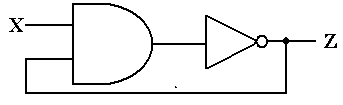This is a bit of an unusual problem, not the sort that I would assign for this course.  I could not resist showing you the solution, once I had worked it out.

In order to analyze this circuit, we must first label the output of the AND gate.  This gives rise to the next version of the figure, with the AND gate output labeled as Y.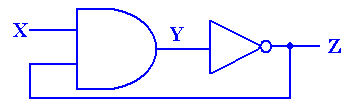Now consider what happens when X = 0.  Recalling that 0·Z = 0, we see that Y = 0 without regard to the value of Z and Z = 1 remains stable.  To pursue the analysis further, we must first look at a slightly different circuit – one without the feedback.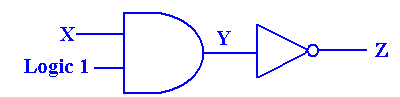When X = 0, Y = 0, and Z = 1.  The following diagram shows what happens just after the moment in time when X becomes 1.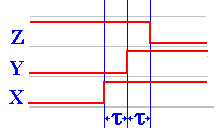At time t after X becomes 1, Y becomes 0.  At time t after Y becomes 0, Z becomes 1.  Thus Z becomes 1 at a time 2t after X becomes 0.  It is this delay of 2t that allows the circuit to display its behavior.

We now analyze the behavior of the full circuit, reproduced here from the previous page.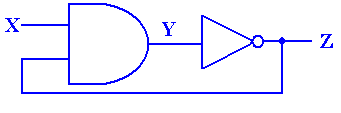The behavior of the circuit is shown in the following diagram.  As noted above, when X = 0, we have Y = 0 (without regard to the value of Z) and Z = 1.  This is a stable situation.  Things change just after X becomes 1.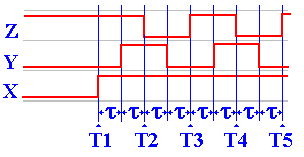1.         At T1, X becomes 1.  Since Z = 1, we have X·Z = 1·1 = 1.  But Y remains at 0.
2.         At T1 + t, Y becomes 1.  Z remains at 1.
3.         At T2 = T1 + 2t, Z becomes 0.  Thus the input to the AND gate are X = 1, Z = 0.
4.         At T2 + t, Y becomes 0.  Z remains at 0.
5.         At T3 = T2 + 2t, Z becomes 1.  Thus the input to the AND gate are X = 1, Z = 1.
6.         At T3 + t, Y becomes 1.  Z remains at 1.
7.         At T4 = T3 + 2t, Z becomes 0.  Thus the input to the AND gate are X = 1, Z = 0.
And so forth.

2.  Use a JK flip-flop to implement an SR flip-flop.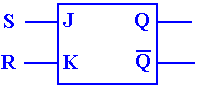This is a trick question.  A JK flip-flop is just like an SR, except that the SR cannot take S = 1 and R = 1.  Just hook up the JK with J = S and K = R and never give it both

J = 1 and K = 1.  This is a really silly questioin.

3.  Use an SR flip-flop and some basic gates (AND, OR, or NOT) to realize a JK flip-flop.

Unlike the previous question, this is a real one.  We must work with the characteristic table of the JK flip-flop and the excitation table of the SR flip-flop.  We use the characteristic table of the JK to specify the desired behavior and the excitation table for the SR flip-flop to determine the input to that flip-flop necessary to make it behave that way.

 J K Q(T+1) 0 0 Q(T) 0 1 0 1 0 1 1 1 Q’(T)

The characteristic table of the JK flip-flop is shown at right.

The main difference between the JK flip-flop and the SR flip-flop is that the JK allows an input J = 1 and K = 1, which causes the state of the flip-flop to change; Q(T+1) = Q’(T).

 Q(T) Q(T+1) S R 0 0 0 d 0 1 1 0 1 0 0 1 1 1 d 0

The excitation table of the SR flip-flop is shown at left.  Note that the transitions between states 0 and 1 are somewhat more constrained that allowed for the JK.

We now rewrite the characteristic table of the JK, explicitly allowing for Q(T) = 0 and
Q(T) = 1.  We then append the appropriate columns of the SR excitation table.

 J K Q(T) Q(T+1) S R 0 0 0 0 0 d 0 0 1 1 d 0 0 1 0 0 0 d 0 1 1 0 0 1 1 0 0 1 1 0 1 0 1 1 d 0 1 1 0 1 1 0 1 1 1 0 0 1

We must now make S and R functions of J, K, and
Q(T).  Note that we cannot use Q(T+1), as this is not a crystal ball circuit.

When J = 0, S matches 0.
When J = 1, S matches Q’(T).  So S = J·Q’(T).

When K = 0, R matches 0.
When K = 1, R matches Q(T).  So R = K·Q(T).

The circuit is shown just below.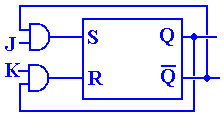There are two ways to produce Boolean expressions for S and R.  These notes will focus on the more intuitive and informal process of pattern matching, which we now try to explain.

Consider first the determination of the expression for S.  It must be given as an expression based only on J, K, and Q(T).  Let’s repeat the table with these four columns only.

 J K Q(T) S 0 0 0 0 0 0 1 d 0 1 0 0 0 1 1 0 1 0 0 1 1 0 1 d 1 1 0 1 1 1 1 0

The goal of any pattern matching is to find an expression that matches the 0’s and 1’s exactly and ignores the “don’t cares”.  In the top half of the table, for which J = 0, note that the number 1 does not appear in the column for S.  This causes us to select one of two choices for S; either S = 0 or S = J.

In the bottom half of the table, for which J = 1, we find both O’s and 1’s in the column for S.  Thus S = 1 is not a match.  We then attempt to find a simple pattern that matches S.  Note that it does not match either K or Q(T), so we must move to more complex.

The key to this match is to note that whenever S = 1, Q(T) = 0 and whenever S = 0, Q(T) = 1.  The best match for the J = 1 half of the table is Q’(T).  Thus the best match for the whole table is S = J·Q’(T).  Other matches include the following canonical form expression.

S = J · K’ · Q’(T) + J · K · Q’(T), which simplifies to the same thing, and
S = J’ · K’ · Q(T) + J · K’ · Q’(T) + J · K’ · Q(T) + J · K · Q’(T), which is silly.

 J K Q(T) R 0 0 0 d 0 0 1 0 1 0 0 0 1 0 1 0 0 1 0 d 0 1 1 1 1 1 0 0 1 1 1 1

To see the best match for the Boolean expression for R, we have rearranged the table so that all the rows for K = 0 come first.  This facilitates seeing the match based on the value of K.

For K = 0, we again see that R = 0 is a good match, as the column for R has no 1’s in the top half of this rearranged table.

For K = 1, we see that Q(T) = 0 whenever R = 0 and Q(T) = 1 whenever R = 1; we do not care about a match for R = d.
Thus the best match for K = 1 is R = Q(T), and the best match
for the whole table is R = K·Q(T).  Again, other matches are possible, but most other candidates are overly complex or just plain silly.

We have now arrived at a set of solutions for the problem.

S = J·Q’(T)

R = K·Q(T)

The only thing left to do is draw the diagram (as shown on the previous page).

A bit later we shall return to a discussion of methods for matching Boolean expressions to columns of 0’s, 1’s, and “don’t cares”.

4.   A set-dominate flip-flop is similar to an SR flip-flop except that input S = 1, R = 1 sets the flip-flop; Q(T + 1) = 1, in stead of being an error condition.  Use one JK flip-flop, and basic gates (AND, OR, NOT) as needed to implement a set-dominate flip-flop; in other words, build a circuit that acts like a set-dominate flip-flop.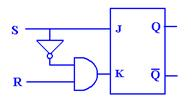The only issue here is again what to do with the input
S = 1, R = 1.  If S = 0, we want J = 0 and K = R. Thus

If S = 1, then we force K = 0.

The following table illustrates.

 S R J = S K = S’·R Q(T+1) 0 0 0 0 0 0 1 0 1 0 1 0 1 0 1 1 1 1 0 1

5    The following diagram is a modified SR flip–flop.  Use a truth table and the characteristic table of an SR flip–flop to determine its characteristic table for inputs X, Y and Z.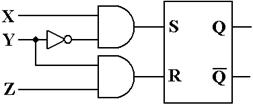ANSWER:         First, note the characteristic table for an SR flip–flop.

 S R Q(t + 1) 0 0 Q(t) 0 1 0 1 0 1 1 1 Error

The important equations, deduced from the diagram above, are as follows:

S = X·Y’                  R = Y·Z

 X Y Z S R Q(t + 1) 0 0 0 0 0 Q(t) 0 0 1 0 0 Q(t) 0 1 0 0 0 Q(t) 0 1 1 0 1 0 1 0 0 1 0 1 1 0 1 1 0 1 1 1 0 0 0 Q(t) 1 1 1 0 1 0

Appendix: The Flip–Flop Tables

The SR Flip–Flop

Characteristic Table

 S R Q(t + 1) 0 0 Q(t) 0 1 0 1 0 1 1 1 ERROR

Excitation Table

 Q(t) Q(t + 1) S R 0 0 0 d 0 1 1 0 1 0 0 1 1 1 d 0

The JK Flip–Flop

Characteristic Table

 J K Q(t + 1) 0 0 Q(t) 0 1 0 1 0 1 1 1(t)

Excitation Table

 Q(t) Q(t + 1) J K 0 0 0 d 0 1 1 d 1 0 d 1 1 1 d 0

The D Flip–Flop

Characteristic Table

 D Q(t + 1) 0 0 1 1

Excitation Equation

D = Q(t + 1)

The T Flip–Flop

Characteristic Table

 T Q(t + 1) 0 Q(t) 1(t)

Excitation Table

 Q(t) Q(t + 1) T 0 0 0 0 1 1 1 0 1 1 1 0

Excitation Equation

T = Q(t) Å Q(t + 1)